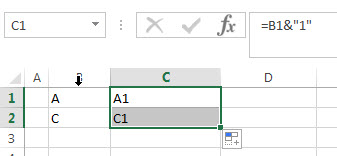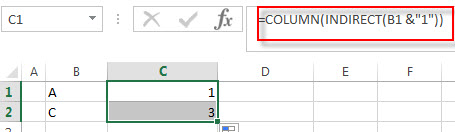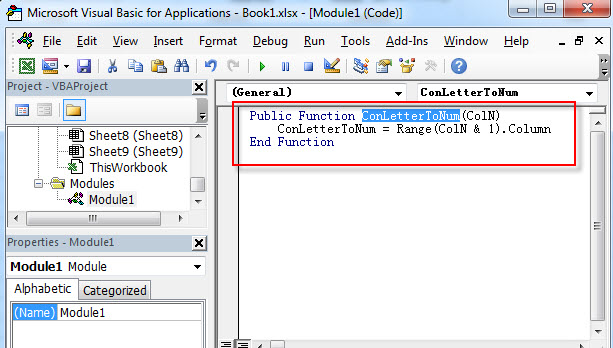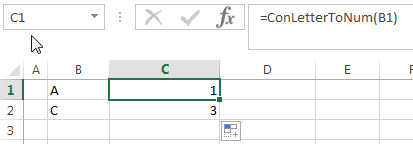# How to convert column letter to number

This post will guide you how to convert a column letter to a column number using an excel formula. And how to write a user defined function to convert column letter to number in excel VBA.

## Convert column letter to number using excel Formula

If you want to convert a column letter to number, you can use a combination of the COLUMN function and the INDIRECT function to create an excel formula.

Firstly, you need to construct a text string of cell reference, such as: B1, then you can use INDIRECT function to transform this text string to a normal cell reference, next, using the COLUMN function to get the column number. So you can write down the following formula:

=COLUMN(INDIRECT(B1 &”1″))

Let’s see how this formula works:

=B1&”1″This formula will join a column number with “1” to construct as a new text string contains column letter. The returned value goes into the INDIRECT function as its argument.

=INDIRECT(B1 &”1″)

The INDIRECT function returns a valid cell reference from a text string returned by the above formula. Then the returned result is passed into the COLUMN function.

=COLUMN(INDIRECT(B1 &”1″))This formula returns the column number for a cell reference that returned by the INDIRECT function. So it returns number 1.

## Convert column number to letter with VBA user defined function

You can also create a new user defined function to convert column number to a column letter in Excel VBA:

1# click on “Visual Basic” command under DEVELOPER Tab.2# then the “Visual Basic Editor” window will appear.

3# click “Insert” ->”Module” to create a new module named4# paste the below VBA code into the code window. Then clicking “Save” button.

```Public Function ConLetterToNum(ColN)
ConLetterToNum = Range(ColN & 1).Column
End Function```5# back to the current worksheet, then enter the below formula in Cell C1:

= ConLetterToNum (B1)### Related Formulas

• Extract text after first comma or space
If you want to get substring after the first comma character from a text string in Cell B1, then you can create a formula based on the MID function and FIND function or SEARCH function ….
• Convert column number to letter
If you want to convert column number to letter, you can use the ADDRESS function to get the absolute reference of one excel cell that contains that column number….

### Related Functions

• Excel COLUMN function
The Excel COLUMN function returns the first column number of the given cell reference.The syntax of the COLUMN function is as below:=COLUMN ([reference])….
• Excel INDIRECT function
The Excel INDIRECT function returns the cell reference based on a text string, such as: type the text string “A2” in B1 cell, it just a text string, so you can use INDIRECT function to convert text string as cell reference….